## Posts

Showing posts from March, 2018

### One More CryptArithmatic ProblemWhat three digits are represented by X, Y, and Z in this addition problem?

XZY

+XYZ
______
YZX

Here is the solution!

### Solution Of One More CryptArithmatic Problem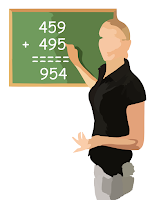What was the problem?

Here is the equation rewritten.

XZY
+XYZ
______
YZX

Let's start with the tens place. Z + Y = Z is there. That means either Y = 0 or 9 if 1 is carry from ones place.

Since Y is at hundred's place it can't be 0. Hence, Y = 9.

At hundred's column, now we have, X + X = 9. That's only possible if X = 4 and carry 1 forwarded from tens place. So X = 4.

Now, finally, at ones place, we have, 9 + Z = 4. Hence, Z must be 5 with carry 1 being forwarded to the next place.

To sum up, X = 4, Y = 9 and Z = 5.

### Who Is The Engineer?On a train, Smith, Robinson, and Jones are the fireman, the brakeman, and the engineer (not necessarily respectively). Also aboard the train are three passengers with the same names, Mr. Smith, Mr. Robinson, and Mr. Jones.

(1) Mr. Robinson is a passenger. He lives in Detroit.
(2) The brakeman lives exactly halfway between Chicago and Detroit.
(3) Mr. Jones is a passenger. He earns exactly \$20,000 per year.
(4) The brakeman’s nearest neighbor, one of the passengers, earns exactly three times as much as the brakeman.
(5) Smith is not a passenger. He beats the fireman in billiards.
(6) The passenger whose name is the same as the brakeman’s lives in Chicago.

Who is the engineer?

### That's Why Smith Is An Engineer!Would you like to read question first?

Let's list all the clues once again here.

(1) Mr. Robinson is a passenger. He lives in Detroit.
(2) The brakeman lives exactly halfway between Chicago and Detroit.
(3) Mr. Jones is a passenger. He earns exactly \$20,000 per year.
(4) The brakeman’s nearest neighbor, one of the passengers, earns exactly three times as much as the brakeman.
(5) Smith is not a passenger. He beats the fireman in billiards.
(6) The passenger whose name is the same as the brakeman’s lives in Chicago.

Since as per (2), the brakeman lives exactly halfway between Chicago and Detroit, locations Chicago or Detroit can't be nearest to him. Hence, the passenger that (4) is suggesting must be nearer to brakeman than Chicago and Detroit.

Now as per (1), Mr. Robinson lives in Detroit, means he is not the nearest to brakeman. Mr.Jones earning is \$20,000/year as per (3), which is not evenly divisible by 3. Hence, the passenger (4) is pointing is not Mr.Jones.

So neither Mr. Robinson

### Unique Audiance For A Fairy Tale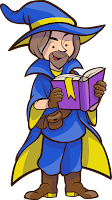A sultan has 14 daughters. He decides to tell every night four of his daughters a fairy tale, but in such a way that every night, there will be another combination of four daughters. How many nights will keep the sultan busy telling fairy tales?

Find number of nights here!

### Nights For Fairy Tales!Story behind the title?

For a moment, let's name all the daughters as A, B, C, D, E.....N.

There are 14 x 13 x 12 x 11 = 24024 combinations of daughters. But in these combinations, lots of combinations are repeated. For example, ABCD combination is same as ABDC or DABC etc. There can be 4 x 3 x 2 x 1 = 24 combinations while considering group of 4 daughters here for example it is A, B, C, D. For these 24 combination we should count only 1 as a unique combination.

Hence for 24024 combinations, we have 24024/24 = 1001 unique combination.

In short, Sultan would be busy for 1001 nights in telling fairy tales to his 14 daughters in unique combinations.

### Numbers For The Letters?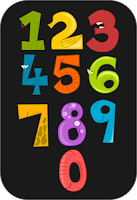A - B = B B * C = A D : B = E C * C = E C + E = A
For which numbers stand A, B, C, D and E

### Correct Numbers For Letters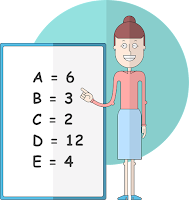What was the question?

Let's first rewrite all equations and number them

1. A - B = B
2. B * C = A 3. D : B = E 4. C * C = E 5. C + E = A
For which numbers stand A, B, C, D and E?
From 1, A = 2B. Putting this in 2 gives,
C = 2.
Putting C = 2 in 4 gives, E = 4.
So from 5, A = C + E = 2 + 4 = 6.
Equation 2 gives, B = A/C = 6/2 = 3.
And equation 3 gives D = B * E = 3 * 4 = 12.

Conclusion : A = 6, B = 3, C = 2, D = 12 and E = 4

### Who Lives Where?There are 4 big houses in my home town.
They are made from these materials: red marbles, green marbles, white marbles and blue marbles.
* Mrs Jennifer's house is somewhere to the left of the green marbles one and the third one along is white marbles.

* Mrs Sharon owns a red marbles house and Mr Cruz does not live at either end, but lives somewhere to the right of the blue marbles house.

* Mr Danny lives in the fourth house, while the first house is not made from red marbles.
Who lives where, and what is their house made from ?

### Owners Of Homes in Home Town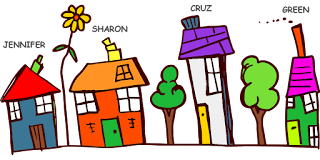What were the clues?

To simplify the process, let's number the houses as 1,2,3,4 and separate clues given.

a. Mrs Jennifer's house is somewhere to the left of the green marbles one.
b. The third one along is white marbles.
c. Mrs Sharon owns a red marbles house
d. Mr Cruz does not live at either end.
e. Mr Cruz lives somewhere to the right of the blue marbles house.
f. Mr Danny lives in the fourth house
g. The first house is not made from red marbles.

As per clues (b) and (g), 1 and 3 aren't made of red marbles. Since Danny is living in 4 as per (f), the red house owned by Mrs. Sharon must be positioned at 2.

Since 2 is already occupied, as per (d), Mr. Cruz must be living at number 3 which is of white marbles according to (b).

According to (a), 4 must be green marbles since otherwise Jennifer wouldn't be somewhere at left. As per (f) this 4 th house is owned by Mr.Danny.

The only house left for Jennifer is blue positioned at 1.

To conclude,

Mrs Jennifer - blue marbles at Numb…

### Mathematical Talk Between Horse And CamelA horse and a camel were carrying boxes on their backs. The horse started complaining to the camel that his load is too heavy.

The camel replied 'Why are you complaining? If you gave me one of your boxes I would have double what you have and if I give you one of my boxes we two would have an even load.'

How many boxes do each of the animal (horse & camel) is carrying ?

Find here the load on each of them!

### Suggestion From Mathematical Talk!What was the talk?

Let's assume C be the number of boxes that camel is carrying and H be that being carried by horse.

As per first part of camel's statement i.e. if you gave me one of your boxes I would have double what you have

C + 1 = 2 (H - 1)

C + 1 = 2H -2

C = 2H - 3 ........(1)

Now in second part (i.e.if I give you one of my boxes we two would have an even load) of camel's statement suggests,

C - 1 = H + 1

C = H + 2

Putting (1) in above,

2H - 3 = H + 2

H = 5

Again putting this value in (1) gives,

C = 2*5 - 3 = 10 - 3 = 7

C = 7.

Horse is carrying 5 boxesand the camel is carrying 7 boxes.

### Fair Distribution Of WaterIn Sahara desert , 3 men found a big 24L Jar is full of water. Since there is shortage of water so they decided to distribute the water among themselves such that they all have equal amounts of it. But they only have a 13L, a 5L and an 11 liter Jar.

How do they do it?

Here is how to do it!

Source

### Equal Distribution Of WaterWhat was the challenge?

1. First pour 24L into 13L and 11L jar.There will be no water in 24L jar.

2. Now pour 13L jar into 5L jar till 5L is filled. So 8L of water will be left in 13L jar.

3. This 8L of water from 13L jar is emptied in empty 24L jar.This will leave 13L jar empty.

4. Now pour 11L water from 11L jar into 13L of jar. There is still space for 2L of water in 13L jar.

5. Pour 5L jar into 13L of jar which had space for 2L only. So 3L of water will be left in 5L of jar.

6. This 3L of water is emptied in 11L jar.

7. A 13L jar full of water is again poured into 5L jar leaving behind 8L of water in it.

8. A 5L jar full of water is finally poured in 11L jar already having 3L of water.

9. This way, 24L, 11L and 13L of jar would have 8L of water each.

### Another CryptArithmatic ProblemReplace letters with numbers assuming numbers can't be repeated.    SEND + MORE ---------- = MONEYProcess of decryption is here!

### Decrypting The CryptArithmatic Problem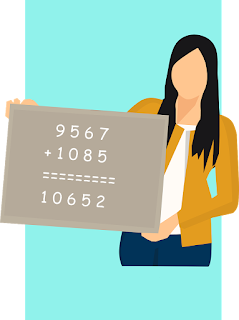What was the problem?

Let's take a look at the equation once again. S E N D + M O R E ----------------- = M O N E YNow letter M must be representing the carry generated & it must be 1. And if M = 1 then S must be 9 or 8 if carry is generated from hundreds place. In any case, O can be either 0 or 1. But can't be 1 as M = 1 hence O = 0.If O = 0 then E + 0 = N i.e. E = N if there is no carry from tens place. Hence, N = E + 1.Let's turn towards tens place now. With no carry from units place N + R = 10 + E .Putting N = E + 1, we get, R = 9. And with carry from units place, we have,1 + N + R = 10 + E, gives us R = 8. Hence either R = 9 and S = 8 or R = 8 or S = 9.For a moment, let's assume R = 9 and S = 8 with no carry from units place, then,  8 E N D + 1 0 9 E ========= 1 0 N E Y For this to be correct, we need carry at thousands place generated from hundreds place. That's only possible if E = 9 and carry is generated from tens placeforwarded to hun…

### Burn The Strings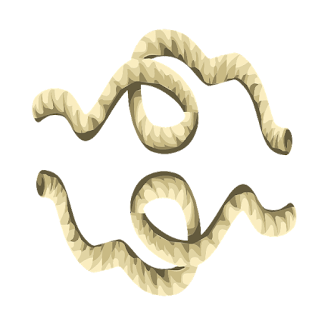You have two strings whose only known property is that when you light one end of either string it takes exactly one hour to burn. The rate at which the strings will burn is constant and strings are identical.

How do you measure 45 minutes?

That's how to measure 45 minutes!

### Timer Using Burning StringsWhat was the challenge?

1. Burn both the ends of 1st string and 1 end of other string.

2. After 30 minutes, 1st string will be burnt out and half of the other string will be burnt out.So far we have counted 30 minutes.

3. Now burn the other end of 2nd end. It will take 15 minute to burnt out totally. This way, we have counted 30 + 15 = 45 minutes using 2 strings.

### "Your Surname Tells My Shirt's Color!"Mr. Yellow, Mr. Black and Mr. Brown, three best friends since kindergarten meet in a function after 5 years. The three of them are wearing either a Yellow, Black or Brown shirt.
After giving each other a friendly hug, Mr. Black says, "Hey, did you notice that we are wearing a different colored shirt than our names!"
The man wearing a Brown shirt said, "Wow I certainly did not notice that but you are right Mr. Black."
Based on this conversation, can you find our who was wearing which colored shirt?

Know color of each person's shirt here!

### Surnames And Shuffling of ShirtsOne thing is sure that Mr.Black is not wearing the BLACK shirt. He is also not wearing BROWN as well as we found the other person wearing theBROWN colored shirt is agreed with the statement made by Mr.BLACK in a conversation. So only color left is the YELLOW & Mr. Black must be wearing that.

Now, the colors left areBROWN and BLACK and persons left are Mr. Yellow and Mr.Brown. Since, each of them wearing different color than his surname, Mr. Brown must be wearing the BLACK shirt. And hence, Mr. Yellow is wearing the BROWN shirt!

To conclude, Mr.Black is wearing YELLOW shirt, Mr. Brown is wearing BLACK shirt & Mr. Yellow must be wearingBROWN shirt.

### Test of an ExaminerFive students - Adam, Cabe, Justin, Michael and Vince appeared for a competitive exam. There were total five questions asked from them from which were twomultiple choicequestions (a, b or c) and three were true/false questions. Their answers are given as follows:
Name I II III IV V

Cabe c b True True False

Adam c c True True True

Justin a c False True True

Michael b a True True False

Vinceb c True False True

Also, no two students got the same number of correct answers. Can you tell the correct answer? Also, what are their individual score?

Know correct answers and scores here!

Source

### Responding To Test of an Examiner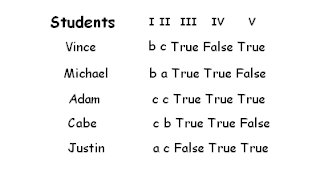What was the test?

There are 2 possibilities of scores & that are either 0,1,2,3,4 or 1,2,3,4,5. First of all, let's arrange students' responses in order like below.

What we notice here is that, there are few responses to same question by different student matching.

For the Question III, only Justin given different answers than other.

Case 1 : If we assume Justin's answer is correct then rest of all are wrong in response to Question III. That means either maximum score in test is 4 or Justin himself has scored 1 to 5.

Let's test that apart from Justin who can have score of 4. If any body other scores 4 then he must share at least 3 similar answers with other (excluding Answer III; refer image below). Only Adam has exact 3 matching responses with Justin.